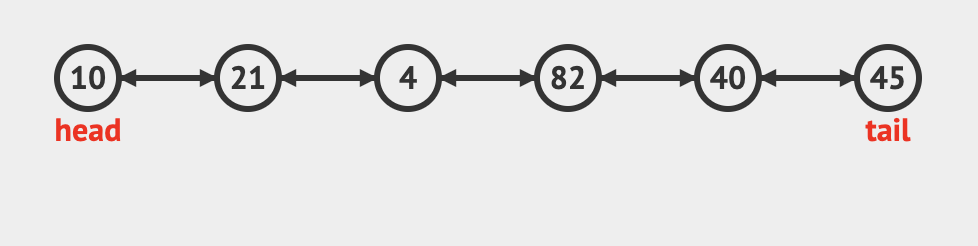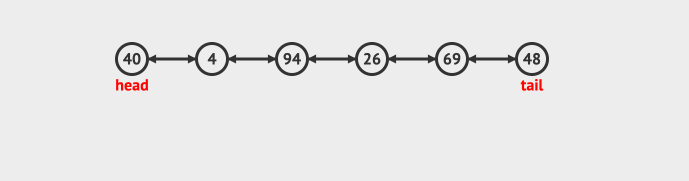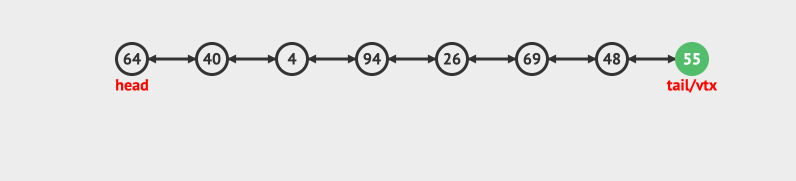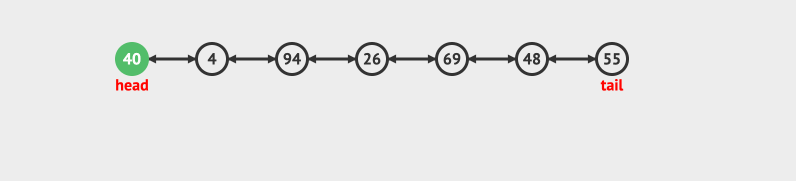# 简介

dequeue指的是双向队列，可以分别从队列的头部插入和获取数据，也可以从队列的尾部插入和获取数据。

# 双向队列的实现

• insertFront(): 从dequeue头部插入数据
• insertLast(): 从dequeue尾部插入数据
• deleteFront(): 从dequeue头部删除数据
• deleteLast(): 从dequeue尾部删除数据1. 在头部插入1. 在尾部插入1. 在头部删除1. 在尾部删除## 双向队列的数组实现

``````//从头部入队列
public void insertFront(int data){
if(isFull()){
System.out.println("Queue is full");
}else{
//从头部插入ArrayDeque
if(rear == -1 ){
}
}
}

//从尾部取数据
public int deleteLast(){
int data;
if(isEmpty()){
System.out.println("Queue is empty");
return -1;
}else{
data= array[rear];
rear = -1;
}else{
rear = (rear + capacity - 1)%capacity;
}
return data;
}
}
``````

## 双向队列的动态数组实现

``````    //因为是循环数组，这里不能做简单的数组拷贝
private void extendQueue(){
int newCapacity= capacity*2;
int[] newArray= new int[newCapacity];
//先全部拷贝
System.arraycopy(array,0,newArray,0,array.length);
newArray[i]= -1;
//拷贝到新的位置
newArray[i+capacity]=array[i];
}
//重置rear的位置
rear = rear +capacity;
//重置capacity和array
capacity=newCapacity;
array=newArray;
}
}
``````

## 双向队列的链表实现

``````public class LinkedListDeQueue {
//rear节点
private Node rearNode;

class Node {
int data;
Node next;
Node prev;
//Node的构造函数
Node(int d) {
data = d;
}
}

public boolean isEmpty(){
}

//从队尾插入
public void insertLast(int data){
Node newNode= new Node(data);
//将rearNode的next指向新插入的节点
if(rearNode !=null){
rearNode.next=newNode;
newNode.prev=rearNode;
}
rearNode=newNode;
}
}

//从队首插入
public void insertFront(int data){
}else{
Node newNode= new Node(data);
}
}

//从队首删除
public int deleteFront(){
int data;
if(isEmpty()){
System.out.println("Queue is empty");
return -1;
}else{
}
return data;
}

//从队尾删除
public int deleteLast(){
int data;
if(isEmpty()){
System.out.println("Queue is empty");
return -1;
}else{
data=rearNode.data;
rearNode=rearNode.prev;
rearNode.next=null;
}
return data;
}

}
``````

learn-algorithm